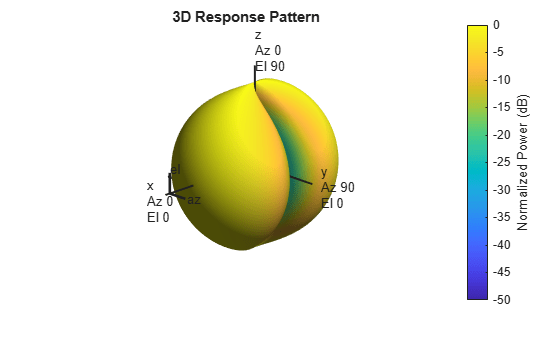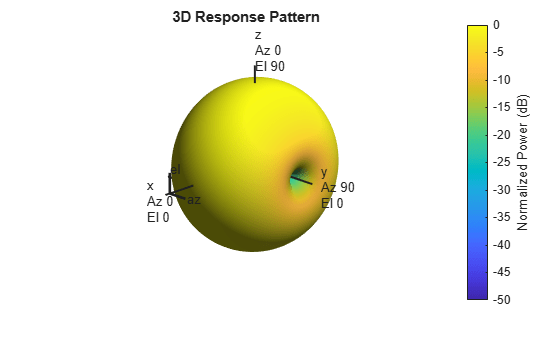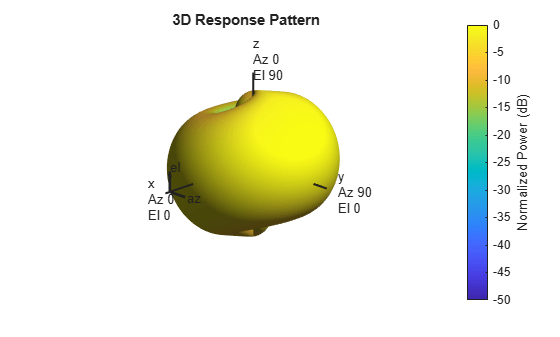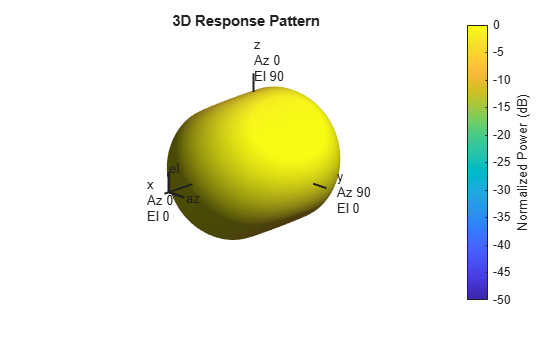# phased.ShortDipoleAntennaElement

Short-dipole antenna element

## Description

The `phased.ShortDipoleAntennaElement` object models a short-dipole antenna element. A short-dipole antenna is a center-fed wire whose length is much shorter than one wavelength. This antenna object only supports polarized fields.

To compute the response of the antenna element for specified directions:

1. Create the `phased.ShortDipoleAntennaElement` object and set its properties.

2. Call the object with arguments, as if it were a function.

To learn more about how System objects work, see What Are System Objects?

## Creation

### Syntax

```antenna = phased.ShortDipoleAntennaElement antenna = phased.ShortDipoleAntennaElement(Name,Value) ```

### Description

`antenna = phased.ShortDipoleAntennaElement` creates the system object, `h`, to model a short-dipole antenna element.

`antenna = phased.ShortDipoleAntennaElement(Name,Value)` creates the system object, `antenna`, with each specified property Name set to the specified Value. You can specify additional name-value pair arguments in any order as (`Name1`,`Value1`,...,`NameN`,`ValueN`).

## Properties

expand all

Unless otherwise indicated, properties are nontunable, which means you cannot change their values after calling the object. Objects lock when you call them, and the `release` function unlocks them.

If a property is tunable, you can change its value at any time.

For more information on changing property values, see System Design in MATLAB Using System Objects.

Antenna operating frequency range specified as a 1-by-2 row vector in the form of `[LowerBound HigherBound]`. This vector defines the frequency range over which the antenna has a response. The antenna element has zero response outside this specified frequency range.

Data Types: `double`

Dipole axis direction, specified as one of `'X'`, `'Y'`, `'Z'`, or `'Custom'`. The dipole axis defines the direction of the dipole current with respect to the local coordinate system. `'X'` specifies a dipole along the x-axis, `'Y'` specifies a dipole along the y-axis, and `'Z'` specifies a dipole along the z-axis. An x-axis or y-axis direction is equivalent to a horizontal dipole and a z-axis direction is equivalent to a vertical dipole. When you set the `AxisDirection` property to `'Custom'`, you can specify the dipole axis using the `CustomAxisDirection` property.

Data Types: `char`

Custom axis direction of the dipole antenna, specified as a real-valued 3-element column vector. Each entry in the vector represents the component of the dipole axis along the x, y, and z axes in the local coordinate system.

#### Dependencies

To enable this property, set the `AxisDirection` property to `'Custom'`.

Data Types: `double`

## Usage

### Syntax

``RESP = antenna(H,FREQ,ANG)``

### Description

example

````RESP = antenna(H,FREQ,ANG)` returns the antenna’s voltage response, `RESP`, at the operating frequencies specified in `FREQ` and in the directions specified in `ANG`. For the short-dipole antenna element object, `RESP` is a MATLAB® `struct` containing two fields, `RESP.H` and `RESP.V`, representing the horizontal and vertical polarization components of the antenna's response. Each field is an M-by-L matrix containing the antenna response at the M angles specified in `ANG` and at the L frequencies specified in `FREQ`.```

### Input Arguments

expand all

Operating frequency of the antenna element, specified as a nonnegative scalar or nonnegative, real-valued 1-by-L row vector. Frequency units are in Hz.

`FREQ` must lie within the range of values specified by the `FrequencyRange` or the `FrequencyVector` property of the element. Otherwise, the element produces no response and the response is returned as `–Inf`. Element objects use the `FrequencyRange` property, except for `phased.CustomAntennaElement`, which uses the `FrequencyVector` property.

Example: `[1e8 2e6]`

Data Types: `double`

Azimuth and elevation angles of the response directions, specified as a real-valued 1-by-M row vector or a real-valued 2-by-M matrix, where M is the number of angular directions. Angle units are in degrees. The azimuth angle must lie in the range –180° to 180°, inclusive. The elevation angle must lie in the range –90° to 90°, inclusive.

• If `ANG` is a 1-by-M vector, each element specifies the azimuth angle of the direction. In this case, the corresponding elevation angle is assumed to be zero.

• If `ANG` is a 2-by-M matrix, each column of the matrix specifies the direction in the form `[azimuth;elevation]`.

The azimuth angle is the angle between the x-axis and the projection of the direction vector onto the xy-plane. This angle is positive when measured from the x-axis toward the y-axis. The elevation angle is the angle between the direction vector and xy-plane. This angle is positive when measured toward the z-axis. See the definition of Azimuth and Elevation Angles.

Example: `[110 125; 15 10]`

Data Types: `double`

### Output Arguments

expand all

Voltage response of antenna element returned as a MATLAB `struct` with fields `RESP.H` and `RESP.V`. Both `RESP.H` and `RESP.V` contain responses for the horizontal and vertical polarization components of the antenna radiation pattern. Both `RESP.H` and `RESP.V` are M-by-L matrices. In these matrices, M represents the number of angles specified in `ANG`, and L represents the number of frequencies specified in `FREQ`.

Data Types: `double`

## Object Functions

To use an object function, specify the System object™ as the first input argument. For example, to release system resources of a System object named `obj`, use this syntax:

`release(obj)`

expand all

 `beamwidth` Compute and display beamwidth of sensor element pattern `directivity` Directivity of antenna or transducer element `isPolarizationCapable` Antenna element polarization capability `pattern` Plot antenna or transducer element directivity and patterns `patternAzimuth` Plot antenna or transducer element directivity and pattern versus azimuth `patternElevation` Plot antenna or transducer element directivity and pattern versus elevation
 `step` Run System object algorithm `release` Release resources and allow changes to System object property values and input characteristics `reset` Reset internal states of System object

## Examples

collapse all

Find the response of a short-dipole antenna element at boresight, (0°,0°), and off boresight, (30°,0°). The antenna operates at 256 MHz.

```antenna = phased.ShortDipoleAntennaElement(... 'FrequencyRange',[100 900]*1e6,'AxisDirection','Y'); ang = [0 30;0 0]; fc = 250e6; resp = antenna(fc,ang)```
```resp = struct with fields: H: [2x1 double] V: [2x1 double] ```

Horizontal response.

`disp(resp.H)`
``` -1.2247 -1.0607 ```

Vertical response.

`disp(resp.V)`
``` 0 0 ```

Specify a short-dipole antenna with the dipole oriented along the y-axis and operating at 250 MHz. Then, plot the 3-D responses for both the horizontal and vertical polarizations.

```antenna = phased.ShortDipoleAntennaElement( ... 'FrequencyRange',[100e6,600e6],'AxisDirection','Y'); fc = 250.0e6;```

Plot the horizontal polarization response.

```pattern(antenna,fc,-180:180,[-90:90],'CoordinateSystem','polar', ... 'Type','powerdb','Polarization','H');```Plot the vertical polarization response.

```pattern(antenna,fc,-180:180,[-90:90],'CoordinateSystem','polar', ... 'Type','powerdb','Polarization','V');```Plot the combined response.

```pattern(antenna,fc,-180:180,[-90:90],'CoordinateSystem','polar',... 'Type','powerdb','Polarization','C');```Specify a short-dipole antenna with the dipole oriented along a custom axis and operating at 250 MHz. Then, plot the 3-D responses for both the horizontal and vertical polarizations.

Create the short-dipole antenna element System object™. An easy way to create a custom axis is to rotate a unit vector using rotation functions.

```v = rotx(30)*rotz(45)*[0;0;1]; antenna = phased.ShortDipoleAntennaElement( ... 'FrequencyRange',[100e6,600e6],'AxisDirection','Custom', ... 'CustomAxisDirection',v);```

Plot the horizontal polarization response.

```fc = 250.0e6; pattern(antenna,fc,-180:180,[-90:90],'CoordinateSystem','polar', ... 'Type','powerdb','Polarization','H');```Plot the vertical polarization response.

```pattern(antenna,fc,-180:180,[-90:90],'CoordinateSystem','polar', ... 'Type','powerdb','Polarization','V');```Plot the combined response.

```pattern(antenna,fc,-180:180,[-90:90],'CoordinateSystem','polar', ... 'Type','powerdb','Polarization','C');```## Algorithms

The total response of a short-dipole antenna element is a combination of its frequency response and spatial response. This System object calculates both responses using nearest neighbor interpolation and then multiplies the responses to form the total response.

 Mott, H., Antennas for Radar and Communications, John Wiley & Sons, 1992.